Import Restraints 9 Equations

Appendix F

Calculating the Cost of a Consumption Basket and CPI change

The cost of the consumption basket of household j in period 0 (year 2015) is  where  is a good,  is its wage-adjusted (real) price, and  is its share in total expenditure in period 0. Share  is obtained from the data (CES), while prices  are obtained from the general equilibrium model. The cost of the same basket in period 1 (year 2020) is . The change in the CPI for a household  between period 1 (year 2020) and period 0 (year 2015) is given by

Consider two alternative scenarios in period 1: (1) the baseline scenario (denoted by superscript b) in which current trade policy remains in place, and (2) a hypothetical scenario (denoted by superscript h) in which trade policy changes. The objective is to compare the effects of the policy change with the baseline development of the economy. Therefore, the expression of interest is

Appendix G

Rouzet and Miroudot (2013) calculate the CT paid on a bilateral country-sector basis between the output from country sector i used in production by country sector j: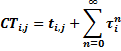In this setup,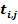represents the direct tariff on imports from country sector i to country sector j; it is an element of the tariff matrix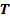and captures the direct tariff incurred at the last border crossing. However, country sector i producers may have already paid tariffs on their inputs, and the producers of those inputs may also have paid tariffs on their inputs, etc. Thus, we assume that up to n countries provide intermediate inputs for the supply chain. The second term captures this indirect effect, where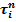is the i-th element of the vector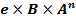, where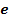is a 1 x J vector of ones;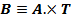., the element-wise multiplication ofand; the requirements matrix from the ICIO table; andis the tariff matrix. Both matrices have the dimensions J x J.

The requirements matrix tells how many cents of input are needed from each country-industry combination to produce one dollar’s worth of output in each producing country-sector. For example, the table will tell us how many cents of manufacturing inputs from Germany are needed to produce one dollar’s worth of agricultural output in Argentina.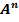is the requirements matrix raised to the power n. In the limit as n goes to infinity, the matrixwill numerically map the entire value chain for each country-industry. For example, it will tell us for each dollar of U.S. agricultural output, how many cents of German chemicals are required. This takes into account both the direct use of chemicals, such as fertilizers, in agriculture, and the indirect use of chemicals used to produce intermediate inputs, such as farming equipment, that are used in agriculture.

In matrix notation, the equation above can be re-written for country-sector pairs as follows:

where TF is a matrix of final tariffs, and e, B and A are as defined above.

This derivation follows Rouzet and Miroudot’s approach for cumulative tariffs embodied in an import. Using the fact that the requirements matrix A is a convergent matrix, the equation for cumulative tariffs can be simplified as follows:

In words, the cumulative tariff is equal to the tariff paid at the last border crossing, TF, and the sum of all tariffs paid on intermediate inputs. The first term represents the non-GSC direct tariff.